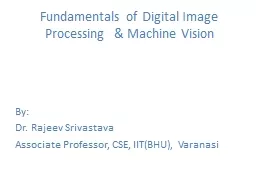# Fundamentals of Digital Image - PowerPoint Presentation#### Fundamentals of Digital Image - Description

Processing amp Machine Vision By Dr Rajeev Srivastava Associate Professor CSE IITBHU Varanasi Fundamentals of Digital Image Processing Applications of image processing Whats an image ID: 430359 Download Presentation

### Tags

digital image set processing image digital processing set color values pixels sampling continuous neighbors adjacent representation denoted signal range

Embed / Share - Fundamentals of Digital Image

## Presentation on theme: "Fundamentals of Digital Image"— Presentation transcript

Slide1

Fundamentals of Digital Image Processing & Machine Vision

By:

Dr. Rajeev Srivastava

Associate Professor, CSE, IIT(BHU), VaranasiSlide2

Fundamentals of Digital Image Processing

• Applications of image processing

• What's an image?

• A simple image model

• Fundamental steps in image processing

• Elements of digital image processing systemsSlide3

• Interest in digital image processing methods

stems from two

principal application areas:

(1)

Improvement

of pictorial information for

human interpretation, and

(2)

Processing

of scene data for autonomous

machine perception.Slide4
Slide5

What's an image?

• A digital image is an image f(

x,y

) that has been

discretized both in spatial coordinates and

brightness.

• The elements of such a digital array are called

image elements or pixels.Slide6

A simple model

The scene is illuminated by a single source.

The

scene reflects radiation towards the camera.

The camera senses it via chemicals on film.Slide7

A simple image model:

To be suitable for computer processing, an image

f(

x,y

)

must be

digitized

both spatially and in

amplitude

.Slide8
Slide9
Slide10

The first thing we have to do, is to obtain signal values from the continuous signal at regular time-intervals.

This

process is known as sampling. The sampling interval is denoted as

and

its reciprocal, the

sampling frequency or

sample-rate is denoted as

,

where

= 1/

.

Slide11

Having defined our sampling interval

,

sampling

just extracts

the signals value at all integer multiples of

Ts

such that our discrete time sequence becomes:

x[n

] = x(n ·

Ts

)

Slide12

At

this point (after sampling), our signal is not yet completely digital because the values x[n]

can still take on any number from a continuous range - that’s why we use the terms discrete-time

signal here

and not digital signal.

Sampling a continuous sinusoidalSlide13
Slide14

QuantizationSlide15
Slide16
Slide17

Now from our continuous range we assign that quantization level which is closest to our actual amplitude: the range 0.05...0.15 maps to 0.1 and so on.

That mapping can be viewed as a piecewise constant function acting on our continuous amplitude variable x.

Characteristic line of a

quantizer

- inputs from a continuous range x are mapped to

discrete levels

Slide18

Quantization NoiseSlide19

Basic Relationships BetweenPixelsSlide20

Neighborhood-

Slide21

Neighborhood-

The four diagonal neighbors of p

(

x,y

)

are given

by,

{(

x+1, y+1), (x+1, y-1), (x-1, y+1), (x-1 ,y-1

)}

This set is denoted by

.

Each

of them are at Euclidean distance

of 1.414

from P.

Slide22

Neighborhood-

Slide23

Neighbors of a pixel

4-neighbors

of a pixel

p are

its

vertical and horizontal

neighbors denoted

by

8-neighbors

of a pixel

p are

its vertical horizontal

and

4 diagonal neighbors

denoted

by

Slide24
Slide25

:

Two pixels

p

and

q

with values from V are

if q is in the set

.

:

Two pixels

p

and

q

with values from V are

if q is in the set

.

Two pixels

p

and

q

with values from V are

,

– q is in

.

– q is in

and the set [

]

is

empty (has

no pixels whose values are from V).

Slide27

Connectivity

It is used to

determine whether the

pixels are

Let V be the set of

gray-level values

used to define

connectivity; then

Two pixels p, q that have

values from

the set V are

:Slide28

4-connected,

if q is in the set

8-connected,

if q is in the set

m-connected,

iff

i. q is in

or

ii. q is in

and the set [

] is empty

Slide29

A

B

CSlide30

Fundamental steps in image processing:

1

.

Image acquisition

: to

acquire

a digital image

2.

Image preprocessing:

to improve the image in

ways that increase the chances for success of the

other processes.

3.

Image segmentation:

to partitions an input image

into its constituent parts or objects.

4.

Image representation:

to convert the input data to

a form suitable for computer processing

.Slide32

Image Representations

Black and white image

single color plane with 2 bits

Grey scale image

single color plane with 8 bits

Color image

three color planes each with 8 bits

RGB, CMY, YIQ, etc.

Indexed color image

single plane that indexes a color table

Compressed images

TIFF, JPEG, BMP, etc.

2gray levels

4 gray levelsSlide33

Digital Image Representation (3 Bit Quantization)Slide34

Color QuantizationExample of 24 bit RGB Image

24-bit Color MonitorSlide35

Image Representation Example

128

135

166

138

190

132

129

255

105

189

167

190

229

213

134

111

138

187

135

190

255

167

213

138

128

138

129

189

229

111

166

132

105

190

134

187

24 bit RGB Representation (uncompressed)

Color PlanesSlide36

Graphical Representation

Shom More....By: cheryl-pisano
Views: 100
Type: Public